Courses

# Digital Electronics MCQ Level - 2

## 10 Questions MCQ Test Topic wise Tests for IIT JAM Physics | Digital Electronics MCQ Level - 2

Description
This mock test of Digital Electronics MCQ Level - 2 for Physics helps you for every Physics entrance exam. This contains 10 Multiple Choice Questions for Physics Digital Electronics MCQ Level - 2 (mcq) to study with solutions a complete question bank. The solved questions answers in this Digital Electronics MCQ Level - 2 quiz give you a good mix of easy questions and tough questions. Physics students definitely take this Digital Electronics MCQ Level - 2 exercise for a better result in the exam. You can find other Digital Electronics MCQ Level - 2 extra questions, long questions & short questions for Physics on EduRev as well by searching above.
QUESTION: 1

### A logic circuit with an output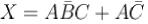consists of :

Solution: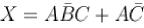can be implemented through the following diagram,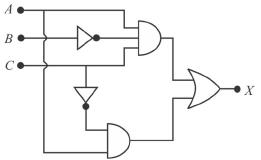Which requires 2 AND gate, 1 OR gate and two inverters The correct answer is: two AND gates, one OR gate and two inverter

QUESTION: 2

### In the given digital logic circuit, A and B form the inputs. The output Y is: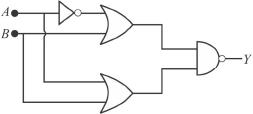Solution: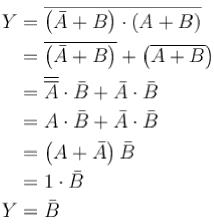The correct answer is: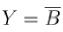QUESTION: 3

### The logic circuit shown in the following figure yields the given truth table.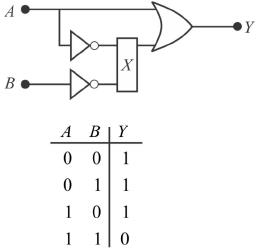The gate X in the diagram is.

Solution:

The truth table of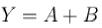If X is NAND gate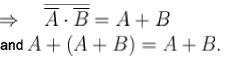Hence, Xis a NAND gate.

QUESTION: 4

A half-adder is a digital circuit with :

Solution: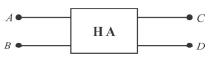The algebraic expression of half-adder are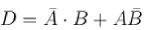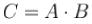The correct answer is: two inputs and two outputs

QUESTION: 5

In the given figure, circuit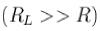performs the operation of :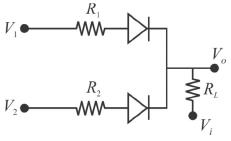Solution:

If either V1 is positive or V2 is positive, V0 be positive, otherwise we get a low output for both V1 and V2 negative. Hence it acts as an OR gate.
The correct answer is: OR gate for a negative logic system

QUESTION: 6

The circuit shown in the figure below functions as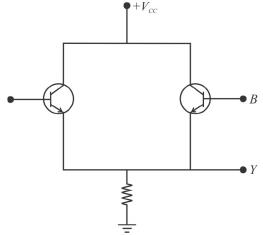Solution:

When A is high and B is low i.e. A = 1, B = 0 transistor A conducts and Y becomes high, i.e. Y = 1. If A = 0 and B = 1; transistor B conducts Y = 1, if A = 1 and B = 1; both transistors conduct. If A = B = 0. None of the transistors conducts hence Y= 0. The true table is shown below.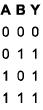The correct answer is: OR gate

QUESTION: 7

The diagram of a logic circuit is given below. The output F is best represented as.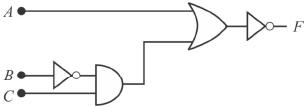Solution:

From the figure,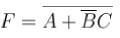The correct answer is: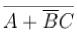QUESTION: 8

Which one of the following gives the output Ffor the logic diagram shown below?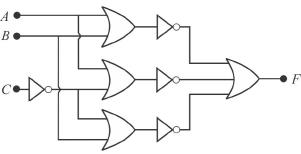Solution: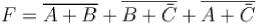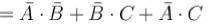The correct answer is: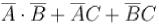QUESTION: 9

The simplest logic gate circuit corresponding to Boolean expression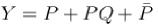is:

Solution: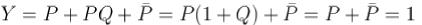The output matches with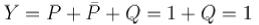Hence, logic for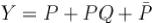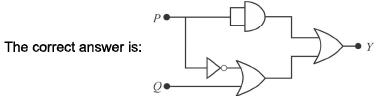QUESTION: 10

The number of bits that a digital computer can process in parallel at a time is called :

Solution:

Word length.
The correct answer is: word length